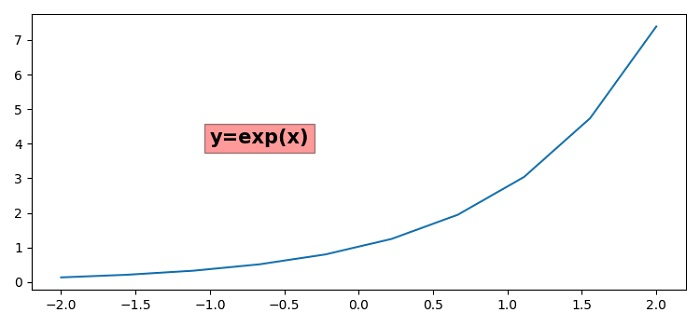# Adjusting Text background transparency in Matplotlib

MatplotlibPythonData Visualization

To adjust text background transparency in matplotlib, we can change the alpha value in the dictionary of bbox with facecolor='red' and alpha='0.4'.

## Steps

• Set the figure size and adjust the padding between and around the subplots.

• Create x and y data points using numpy.

• Plot x and y data points using plot() method.

• Now use text() method to adjust the text background with fontdict and bbox dictionaries at x=-1.0 and y=4.0.

• To display the figure, use show() method.

## Example

import numpy as np
from matplotlib import pyplot as plt
plt.rcParams["figure.figsize"] = [7.50, 3.50]
plt.rcParams["figure.autolayout"] = True
x = np.linspace(-2, 2, 10)
y = np.exp(x)
plt.plot(x, y)
plt.text(-1.0, 4.0, 'y=exp(x)', fontdict=dict(fontsize=15, fontweight='bold'), bbox=dict(facecolor='red', alpha=0.4, edgecolor='black'))
plt.show()

## Output